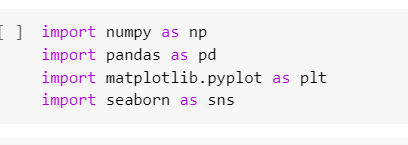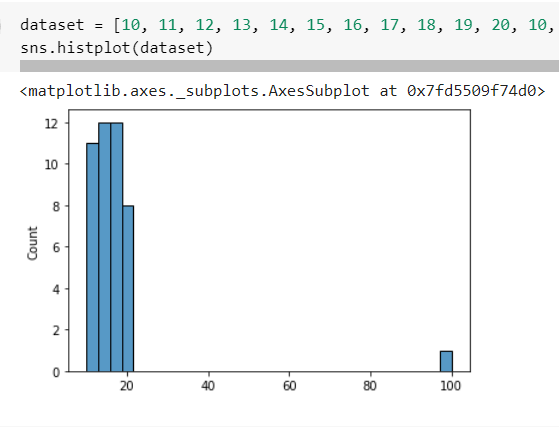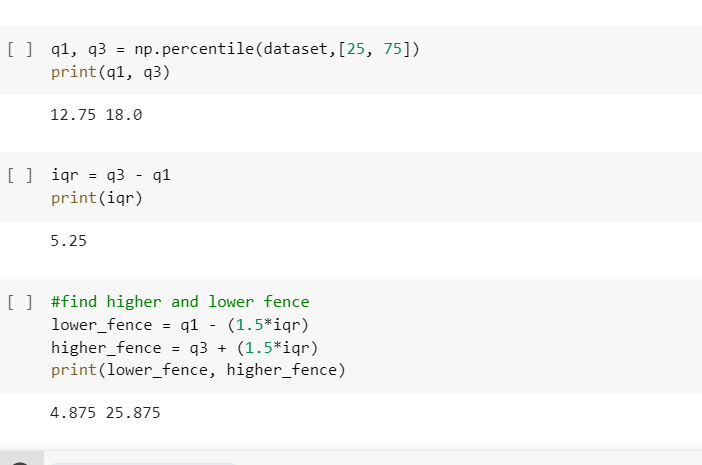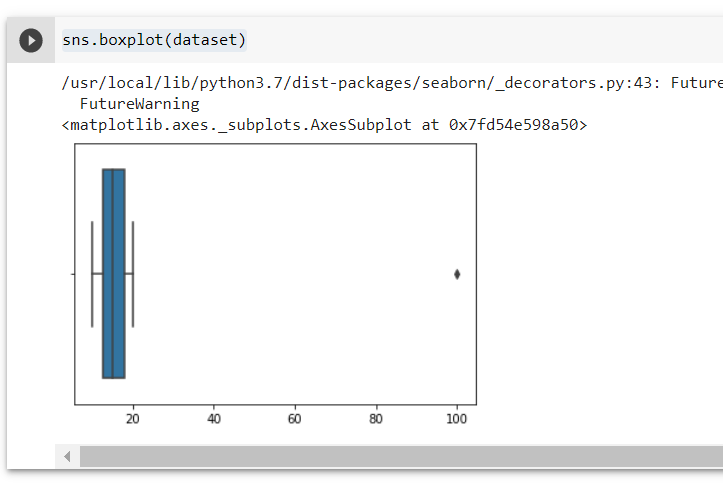# Use Python to Find the InterQuartile Range of a Dataset

## A tutorial on finding the interquartile range of a dataset using Python.In statistics, the interquartile range tells the middle half of the distribution of a dataset. Quartiles segment any distribution that is ordered from low to high into four equal parts. The interquartile range, or IQR, contains the second and third quartiles, or the middle half of the dataset.

There are four steps in defining the IQR, which are listed below:

1. Sort the data.
2. Calculate Q1 and Q3.
3. IQR = Q3 — Q1.
4. Find the lower fence, being Q1 — (1.5*IQR).
5. Find the upper fence, being Q3 + (1.5*IQR).

I created a script using Google Colab, which is a free online Jupyter Notebook that allows people to write programs in Python.

Once I created the Jupyter Notebook, I imported the libraries I would need to run it. The libraries I imported were:-

1. Numpy to carry out mathematical calculations and create NumPy arrays,
2. Pandas to create and manipulate dataframes,
3. Matplotlib to plot the data points onto a graph, and
4. Seaborn to carry out statistical graphical functions.I then created a dataset, ensuring it had an outlier in it:-Once the dataset was created and plotted on a graph using seaborn, I used NumPy to calculate the interquartile range:-

1. I used NumPy's percentile to compute the first and third quartiles.
2. I calculated the interquartile range, iqr, by subtracting q1 from q3.
3. I then calculated the lower and upper fences, which took into consideration the outlier.Lastly, I used seaborn to create a box plot of the dataset, noting that the outlier is at the far right of the plot:-The iqr is very important in statistics because it can be useful in removing outliers from a dataset.

I have prepared a code review for this post, which can be viewed here:- https://www.youtube.com/watch?v=p1xQoumao8g